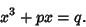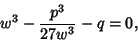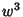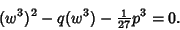## Vieta's Substitution

The substitution of(1)

into the standard form Cubic Equation(2)

The result reduces the cubic to the equation(3)

which is easily turned into a Quadratic Equation inby multiplying through byto obtain(4)